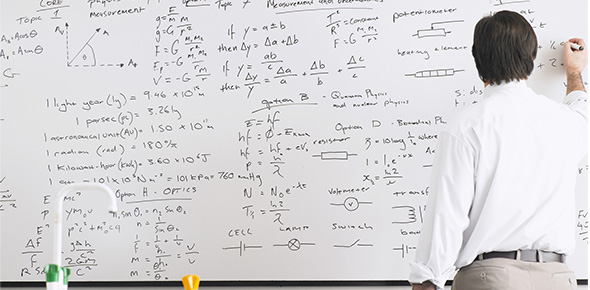# Literal Equations Quiz Questions

5 Questions | Total Attempts: 83SettingsThis quiz has five questions and should be completed in five minutes.

• 1.
• A.

K=A-2c

• B.

K=2-A/c

• C.

K=2Ac

• D.

K=A/2c

• E.

K=A/(2c)

• 2.
• A.

Q=3(R+H)

• B.

Q=1/3R-H

• C.

Q=3R-H

• D.

Q=3(R-H)

• E.

Q=1/3R+H

• 3.
• A.

M=(B-T)/2

• B.

M=T/2+B

• C.

M=2T+B

• D.

M=(T+B)/2

• E.

M=T+B/2

• 4.
• A.

A=2s+2b

• B.

A=2s-b

• C.

A=b-2s

• D.

A=1/2s-1/2b

• E.

A=2s+b

• 5.
• A.

Y=x-3/2

• B.

Y=-2x-3

• C.

Y=-x-3/2

• D.

Y=-2x+3/2

• E.

Y=-2x-3/2

Related TopicsBack to top